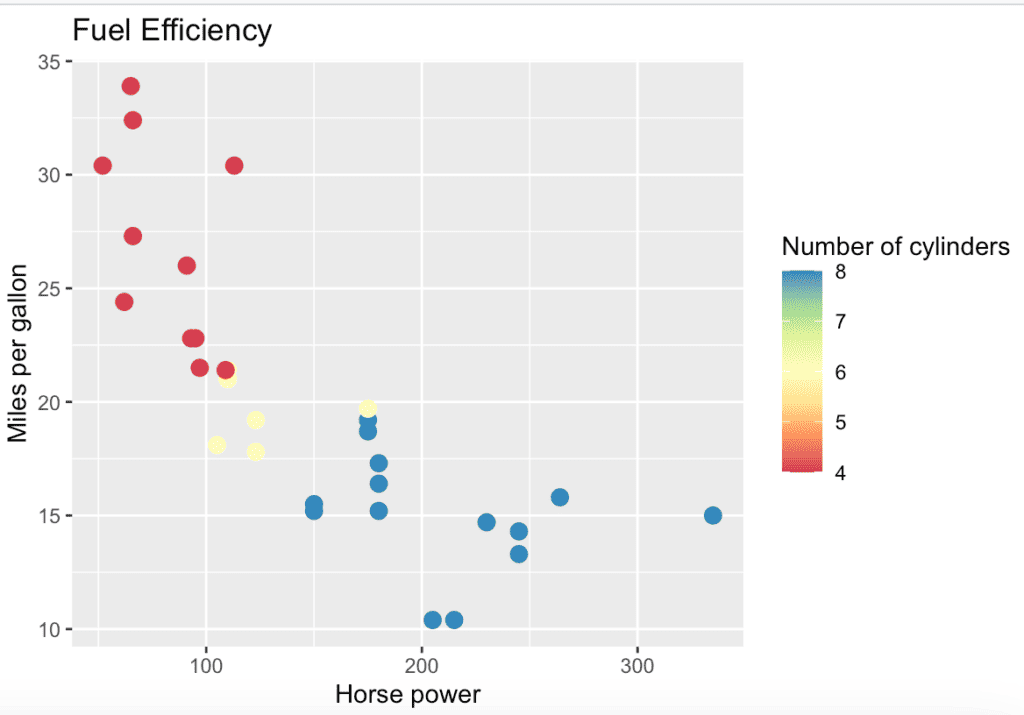# R scale_colour_distiller() Function

The scale_colour_distiller() function is used to apply a color scale to the color aesthetic of a plot. It uses a color scale generated using a distiller color scheme, a type of color scheme that emphasizes perceptual uniformity and is commonly used for data visualization.

## Syntax

``````scale_colour_distiller(
type = "seq",
palette = 1,
direction = -1,
values = NULL,
space = "Lab",
na.value = "grey50",
guide = "colourbar",
aesthetics = "colour"
)``````

## Parameters

1. type: It is one of “seq” (sequential), “div” (diverging), or “qual” (qualitative)
2. palette: If a string will use that named palette. The list of available palettes can be found in the Palettes section.
3. direction: It sets the order of colors in the scale. If 1, the default colors are as output by RColorBrewer::brewer.pal(). If -1, the order of colors is reversed.
4. aesthetics: The character string or vector of character strings listing the name(s) of the aesthetic(s) that this scale works with.
5. guide: Type of legend. Use “colourbar” for continuous color bar or “legend” for discrete color legend.
6. na.value: It is a color for missing values.
7. values: If colors are not evenly positioned along the gradient, this vector gives the position (between 0 and 1) for each color in the colors vector.
8. space: It is a color space in which to calculate the gradient. Must be “Lab” – other values are deprecated.

## Example

``````library(ggplot2)

# Create a scatterplot of car data
ggplot(mtcars, aes(x = hp, y = mpg, color = cyl)) +
geom_point(size = 3) +
labs(
title = "Fuel Efficiency",
x = "Horse power", y = "Miles per gallon",
color = "Vehicle Class"
) +
scale_colour_distiller(
palette = "Spectral", direction = 1,
name = "Number of cylinders"
)``````

OutputThat’s it.